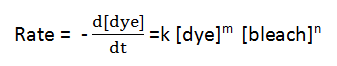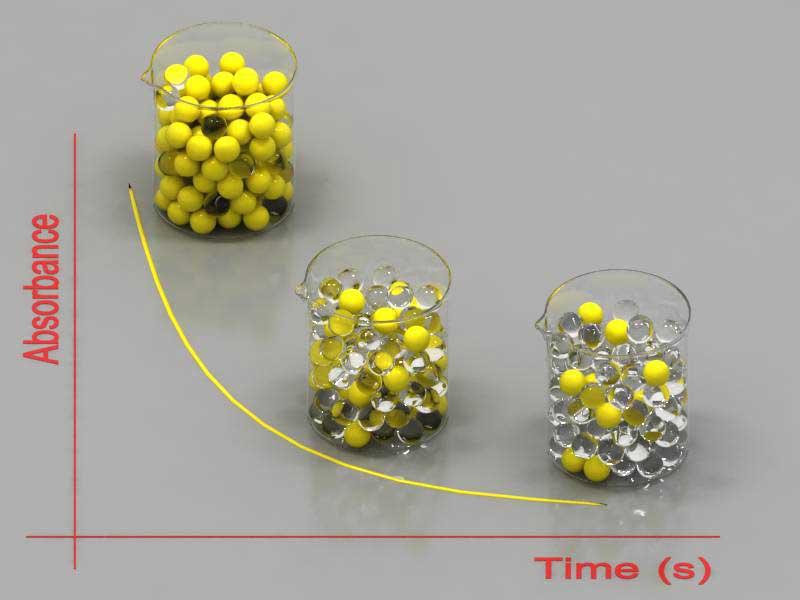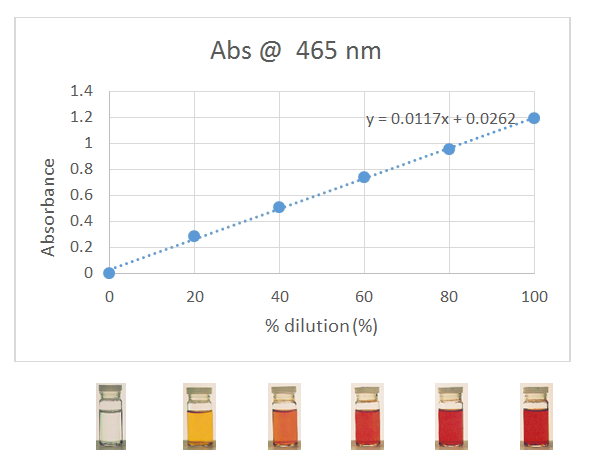# Experiment 11

-Introduction

-Background Step 1

-Background Step 2

-Step 1: Making a kinetic trace

-Step 2: Getting information from a kinetic trace

-Step 2: Determining the pseudo rate constant k'

-Step 3: Determine the effect of bleach on the reaction

-Step 4: Putting it all together

-Step 4: Calculating Instantaneous Reaction rates

-Feedback Form

Experiment 11 - Kinetics of Food Dyes >> Introduction

 Next >

# Experiment 11 - Kinetics of Food Dyes

## Introduction

Reactivity and kinetic stability of chemical systems play an important role in the chemistry of the world around us: in industry, in medicine, in engineering, and in the environment, to name a few. A reaction can be allowed by thermodynamics, but if it takes years or centuries to occur, the reaction will not be of much use. In addition to these practical aspects, measuring reaction rates often gives us insight into how it occurs, revealing the mechanism of complex chemical processes. The following movie shows what happens when a dye reacts with bleach.

In this experiment the goal is to determine the "rate law" for the reaction of a food dye with bleach. The rate law summarizes experimental information in a concise manner: once we have the rate law, we can predict the rate of the reaction for a wide range of experimental conditions. The rate law has this form:and contains two types of information:

• The order of the reaction, n and m above, with respect to the concentrations of the reactants. The rate is proportional to the concentration raised to the "order". For first order, the rate is proportional to concentration raised to the first power, so doubling the concentration doubles the rate. For second order, the rate is proportional to the concentration raised to the second power, so doubling the concentration increases the rate by a factor of four.

• The rate constant, k above, is the proportionality constant. We need the rate constant if we want to calculate the rate instead of just understand how the rate changes when we change the concentration.

To determine the rate law, we will measure the rate under different conditions. The goal of this tutorial is to get you ready to carry out these experiments in the laboratory.

The conditions we will be changing are the concentrations of the reactants, for instance, we will double the concentration of the bleach. We will run the reaction twice. Both times, we will start with 10 mL of a Yellow 6 dye solution. The first time, we will mix in 0.30 mL of 0.090 M NaOCl (household bleach). The second time, we will use 0.30 mL of bleach that is twice as concentrated, i.e. 0.180 M NaOCl. Which reaction do you think will go faster? Check out the following movie and see if you are correct.

How did you tell which reaction went faster? You probably watched how rapidly the colour faded. This works because, as the dye reacts with the bleach, its concentration decreases and the colour fades. We will take advantage of this in the lab by using a spectrometer to precisely measure the intensity of the colour. The quantity that measures "intensity of the colour" is the "absorbance" of the solution. Using Beer's Law, we can convert absorbance values into concentrations. This is great for chemical species that impart colour to a solution. But what do we do for the bleach, which is colourless? We need to find a way to monitor the concentration of the bleach indirectly. This indirect measurement is a research project you will design for the lab.

To begin, let's take a look at the overall experimental strategy as it is shown schematically below. We will then dive in and look at each step in detail.

## Step 1: Follow the disappearance of the dye by measuring the absorbance of the dye during the reaction.

Making a "kinetic trace": Follow the rate of disappearance of the coloured reactant by colour spectroscopy.

## Step 2: Getting information from a kinetic trace.Measuring the absorbance of the dye during its reaction with bleach is expressed graphically on the screen as the spectrophotometer takes a reading of absorbance every second or so. We will learn how the analysis of this graph (it is called a kinetic trace) can give us an insight into the rate of reaction.

## Step 3: Design a research project to determine the effect of bleach on the rate of reaction.

Once we have determined what happens to the dye during the reaction, we need to find a way to monitor the bleach indirectly, since it is colourless and its concentration cannot be monitored by the spectrophotometer.

 What will happen to the RATE when I make a change?

## Step 4: Putting it all together

We will be assembling the rate law expression and calculating instantaneous reaction rates. To do this, we will need to know the concentrations of dye during the reaction. In the laboratory, we measure absorbance. Beer's Law tells us that absorbance is proportional to concentration, [dye]. And so if we determine that proportionality, we can convert from our measured absorbances to [dye].Beer's Law Calibration

Step 1

Action: We monitor the absorbance of the dye over time as it reacts with bleach. (This plot of absorbance versus time is called a kinetic trace).

Result: We obtain a kinetic trace for the reaction. We can use this data to determine the reaction order with respect to the dye.

Step 2

Action: We consider three different forms for the rate law, corresponding to the rate being zeroth, first, or second order in the concentration of dye. We use a graphical approach to determine which rate law applies to our kinetic trace, which in turn tells us the order of the reaction with respect to dye.

Result: We determine the reaction order in dye.

Step 3

Action: Based on the knowledge gained in the previous steps, we ask research questions, create hypotheses and design an experiment to determine how changes in the concentration of bleach will change the rate of the reaction.

Result: We perform an experiment which will allow us to determine the reaction order with respect to bleach.

Step 4

Action: The kinetic traces we obtain in steps 1 through 3 give absorbance of the solution versus time. To get the rate law, we need to convert from absorbance to dye concentration. This is not difficult, because absorbance is proportional to dye concentration, via Beer's Law. All we need to do is determine this relationship. To do this, we prepare a set of dilutions of the standard dye (its concentration is written on the bottle). We then measure the absorbance of each diluted solution at the analytical wavelength.

Result: We obtain a calibration plot of absorbance versus concentration at the analytical wavelength for the standard dye. We can use the slope of this plot to convert absorbance readings to concentrations in the kinetic trace.

We use the concentrations in the kinetics experiment to assemble the rate law and calculate instantaneous reaction rates.

The following pages contain tutors to assist you with performing kinetics experiments and the calculations required in the above steps.

 Next >